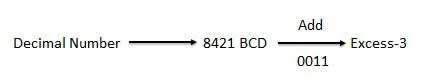# BCD to Excess-3 code converter using logic gates

<Back

## Aim

To study and Verify the BCD to Excess-3 code converter using logic gates.

## Learning Objectives

1. To understand the behavior and demonstrate the Implementation of BCD to Excess-3 code converter using logic gates.
2. To apply knowledge of the fundamental gates to create truth tables.
3. To develop digital circuit building and troubleshooting skills.
4. To understand key elements of TTL logic specification or datasheets.

## IC Used

For BCD to Excess-3 code converter using logic gates:
IC NumberIC Name
74LS04Hex Inverting Gates

## Procedure

1. Place the IC on IC Trainer Kit.
2. Connect VCC and ground to respective pins of IC Trainer Kit.
3. Implement the circuit as shown in the circuit diagram.
4. Connect the inputs to the input switches provided in the IC Trainer Kit.
5. Connect the outputs to the switches of O/P LEDs
6. Apply various combinations of inputs according to the truth table and observe the condition of LEDs.
7. Note down the corresponding output readings for various combinations of inputs.
8. Power Off Trainer Kit, disconnect all the wire connections and remove IC's from IC-Base.

## Theory

Binary Coded Decimal (BCD) Code -​ Digital numbers are represented, stored and transmitted as group of binary bits. This group is also called as binary code decimals. Weighted binary codes are those binary codes which obey the positional weight principle. Each position of the number represents a specific weight. Several systems of the codes are used to express the decimal digits 0 through 9. In these codes each decimal digit is represented by a group of four bits.

In this code each decimal digit is represented by a 4-bit binary number. BCD is a way to express each of the decimal digits with a binary code. In the BCD, with four bits we can represent sixteen numbers (0000 to 1111). But in BCD code only first ten of these are used (0000 to 1001). The remaining six code combinations i.e. 1010 to 1111 are invalid in BCD.

• It is very similar to decimal system.
• We need to remember binary equivalent of decimal numbers 0 to 9 only.

• The addition and subtraction of BCD have different rules.
• The BCD arithmetic is little more complicated.
• BCD needs more number of bits than binary to represent the decimal number. So BCD is less efficient than binary.
• Excess-3 Code - The Excess-3 code is also called as XS-3 code. It is non-weighted code used to express decimal numbers. The Excess-3 code words are derived from the 8421 BCD code words adding (0011)2 or (3)10 to each code word in 8421. The excess-3 codes are obtained as follows -BCD to Excess-3 Code Converter - Excess-3 binary code is an unweighted self-complementary BCD code. Self-Complementary property means that the 1’s complement of an excess-3 number is the excess-3 code of the 9’s complement of the corresponding decimal number. This property is useful since a decimal number can be nines’ complemented (for subtraction) as easily as a binary number can be ones’ complemented; just by inverting all bits.

As is clear by the name, a BCD digit can be converted to it’s corresponding Excess-3 code by simply adding 3 to it.

## Block Diagram## Precautions

1. Make the connections according to the IC pin diagram.
2. The connections should be tight on trainer kit.
3. The Vcc and ground should be applied carefully at the specified pin only.

## Conclusion

We have learned the Implementation of BCD to Excess-3 code converter using logic gates.